Home | | Physics 11th std | Long Questions and Answer

## Chapter: 11th Physics : UNIT 2 : Kinematics

Physics : Kinematics : Book Back Important Questions, Answers, Solutions : Long Questions and Answer

Physics : Kinematics

1. Explain in detail the triangle law of addition.● The two vectors are represented by two adjacent sides of a triangle taken in the same order. Then the resultant is given by the third side of the triangle.

● The head of the first vectoris connected to the tail of second vector● Let θ is the angle betweenand● R is the resultant vector connecting the tail of the first vectorto the head of the second vector.

●1) Magnitude of resultant Vector :● Consider the triangle ABN which is obtained by extending the side OA to ON.

● ABN is a right angled triangle.

cos θ = AN / B

AN = B cos θ        ……… (1)

cos θ = BN / B

AN = B cos θ        ……… (2)

For ∆ OBN, OB2 = ON2 + BN2        ……… (3)

R2 = (A+B cosθ)2 + (B sinθ)2

R2 = A2 + B2 cos2θ + 2AB cos + B2sin2θ

R2 = A2 + B2 (cos2θ sin2θ) + 2AB cosθ

R2 = A2 + B2 + 2AB cosθ

R = √[A2 + B2 + 2AB cosθ]  ………… (4)

2. Direction of resultant Vectors :

If θ is the angle betweenand, then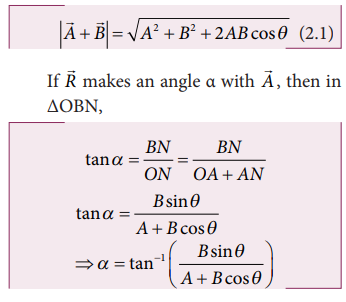In ∆OBN

tan α = BN / ON = BN / (OA + AN)

tan α = Bsinθ / (A + B cosθ)

α = tan-1 (Bsinθ / [A + B cosθ])       ……….(6)

2. Discuss the properties of scalar and vector products.

Properties of scalar Product:

(i) The product quantity.is always a scalar. It is positive if the angle between the vectors is acute and negative if the angle between them is obtuse.

(ii) The scalar product is commutative.=.(iii) The vectors obey distributive law i,e,(iv) The angle between the vectors(v) The scalar product of two vectors will be maximum when cosθ = 1 i.e. θ = 0° When the vectors are parallel (.)max = AB

(vi) The scalar product of two vectors will be minimum when cosθ = −1 i.e. θ = 180° .

()mini = −AB when the vectors are anti parallel.

vii) If the vectorsandare perpendicular to each other then their scalar product.= 0. Then the vectorsandare said to be mutually orthogonal.

viii) The scalar product of a vector with itself is termed as self dot product and is given byThe magnitude (or) norm of the vectorsis || = A = √{.}

ix) In the case of a unit vector n^x) In the case of orthogonal unit vectors i^, j^ and k^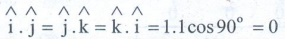Properties of vector product:3. Derive the kinematic equations of motion for constant acceleration.

● Consider an object moving in a straight line with uniform constant acceleration.

● Let u be the velocity of the object at time t = 0.

● v be the velocity of the body at a time t.

Velocity - time relation :

i) The acceleration of the body at any instant is given by the first derivative of velocity with respect to time.

a = dv / dt (or) dv = a.dt                 ……. (1)

Integrating on both sides[v]uv = a [t]0t

v – u = at

v = u + at        ……..(2)

Displacement - time relation :

ii) The velocity of the body is given by the first derivative of the displacement with time.

v = ds / dt

ds = v.dt              …………….(3)

since v = u+at

ds = (u+at) dt          ……………. (4)

intergrating on both sidess = ut + ½ at2           …………(5)

Velocity - displacement relation:

iii) The acceleration is given by the first derivative of velocity with respect to time.

a = dv / dt = dv/ds . ds/dt = v . dv/ds           (ds / dt = v)

a = ½ dv2/ds

ds = 1/2a d(v2)         ………..(6)

Integrating on both sides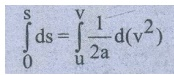s = 1/2a (v2 – u2)

v2 = u2 + 2as      …………(7)

From equation

at = v - u        ……………. (8)

Substitute equation (8) in equation (5) we get,

s = ut+ ½ (v-u) t

s = [(u+v)t] / 2          …………(9)

Kinematic equations,

v = u+at

s = ut+ ½ at2

v2 = u2 + 2as

s = [(u+v)t ] / 2

4. Derive the equations of motion for a particle (a) falling vertically (b) projected vertically

i) A body falling vertically from a height h:

● Consider an object of mass in falling from a height h.

● Let us choose the downward direction as positive y axis.● The object experience acceleration 'g' due to granty which is constant near the surface of the earth. The accelerationBy comparing the components we get, ax =0, az =0, ay = g

ay = a = g

● If the particle is thrown with initial velocity u downward which is in negative y axis.

● Then velocity and position of the particle at any time t is given by,

v = u + gt  ……....... (1)

y = ut + ½ gt2       .......... (2)

The square of the speed of the particle is

v2 = u2 + 2gy     ......... (3)

Suppose the particle starts from rest

Then u = 0

v = gt        .......... (4)

y = ½ gt2         .......... (5)

v2 = 2gy      .......... (6)

b) A body thrown vertically upwards :

● An object of mass m thrown vertically up-wards with an initial velocity u.

● The acceleration a = −g and g points towards the negative y axis.● The Kinematic equations for this motion are

v = u − gt  ………. (7)

s = ut – ½ gt2    ………. (8)

v2 = u2 -2gy

5. Derive the equation of motion, range and maximum height reached by the particle thrown at an oblique angle θ with respect to the horizontal direction.

● Consider an object thrown with initial velocityu at an angle θ with the horizontal.

ThenWhere ux = u cos θ is the horizontal component.

uy = u sinθ is the vertical component of velocity.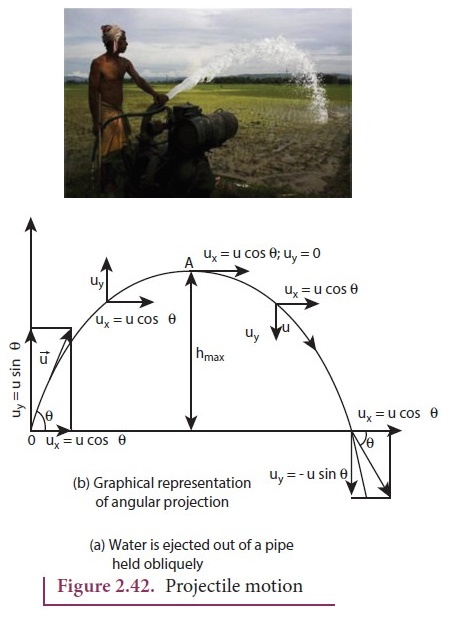After the time t1 the velocity along horizontal motion

vx = u + ax

t = ux = u cosθ

The horizontal distance is

sx = uxt + ½axt2

Here sx = x, ux = u cosθ, ax = 0

x = u cosθ.t

t = x / ucosθ        ………..(1)

For vertical motion vy = uy + ayt

Here uy = u sinθ, ay = −g

vy = u sinθ – gt      ………(2)

The vertical distance travelled by the projectile is

sy = uyt + ½ ay t2

Here sy = y, uy = u sinθ, ax = −g

than y = u sinθ.t – ½ gt2    ………(3)

Substitute the 't' value from the equation (1) in equation (3), we have

y = u sinθ [ x / ucosθ ]  –  ½ g [ x2 / u2 cos2θ ]   ………(4)

The path followed by the projectile is an inverted parabola.

Maximum (hmax )

The maximum vertical distance travelled by projectile during its journey is called maximum height.

For vertical part of motion,

v2y = u2y + 2ayS

Here, uy = u sinθ, ay = −g, s = hmax ; vy = 0

O = u2sin2 θ − 2ghmax

hmax = u2sin2θ / 2g…………(5)

Horizontal Range (R):

The maximum horizontal distance between the point of projection and the point on the horizontal plane where the projectile hits the ground is called horizontal range (R).

Range R = Horizontal compoent of velocity × time of flight

R = u cosθ × Tf

R = ucosθ × [2u sinθ / g] = [ 2u2 sinθ cosθ ] / g

R = [u2 sin2θ] / g……………… (6)

The maximum possible range is reached when sin2θ = l,

When 2θ = π /2 (or) θ = π /4

Rmax = u2 / g…………..(7)

6. Derive the expression for centripetal acceleration.

● The acceleration acting on an object towards the center of the circle in a uniform circular motion is known as centripetal acceleration.

● The centripetal acceleration is derived from a simple relationship between position and velocity vector.● The directions of position and velocity vectors shift through the same angle θ in small time ∆t as shown in the above figure

● For uniform circular motion r = || = |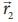| and  v = || = |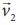|

● If the particle moves from position vectorto● The displacement● Then change in velocitythen ∆r/r = - ∆v/v = θ        …………. (1)

Here negative sign implies that ∆v points radially inward, towards the centre of the circle.

∆v = −v (∆r / r)

a = ∆v / ∆t = v/r (∆r / ∆t) = −v2 / rFor uniform circular motion v = rω

Then centripetal acceleration a = −v2 / r = - ω2r7. Derive the expression for total acceleration in the non uniform circular motion.

● If the speed of the object in circular motion is not constant then it is called non-uniform motion.

Example : The bob attached to a string moves in vertical circle.

● The speed of the bob is not the same at all time.

● The speed is not same in circular motion, the particle will have both centripetal and tangetial acceleration.

The centripetal acceleration is v2/r

The magnitude of resultant acceleration is

aR = √ [ at2 + (v2 / r)2 ]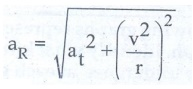The resultant acceleration makes an angle θ with the radius vector.

The angle is given by tan θ = at / (v2 / r)Tags : Kinematics | Physics , 11th Physics : UNIT 2 : Kinematics
Study Material, Lecturing Notes, Assignment, Reference, Wiki description explanation, brief detail
11th Physics : UNIT 2 : Kinematics : Long Questions and Answer | Kinematics | Physics